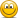# Errors Found in Study Materials P2.T8. Liquidity and Treasury Risk (OLD thread)

Status
Not open for further replies.

#### Nicole Seaman

##### Director of FRM Operations
Staff member
Subscriber
Please use this thread to let David and I know about any errors, missing/broken links, etc. that you find in the materials that are published in the study planner under P2.T8. Liquidity and Treasury Risk. This will keep our forum much more organized. We appreciate your cooperation!PLEASE NOTE: Our Practice Question sets already have links to their specific forum threads where you can post about any errors that you find. The new forum threads are for any other materials (notes, spreadsheets, videos,etc.) where you might find errors.

Information needed for us to correct errors:

• Page number
• Error

#### akash3009

##### New Member
Hi,

I think the example for the cost of liquidation is not correct. This is from reading P2.T8 page 4 and 5. If we use the formula of liquidity price as s*a/2 then the cost of liquidity should be as follows:

position 1 : (100.50-100)/100.25 * (100 mil /2) * 100.25 = 24999994.125
position 2 : (20.10 - 20)/20.05 * (200 mil / 2) * 20.05 = 9999999.855

So total cost of liquidation = 34,999,993.98 and not 105,011,875 as in the text.

Please suggest if I got this wrong.

Regards,
Akash Dhenki

#### evelyn.peng

##### Active Member
R44, Hull, Chapter 24
page 14 out of 22 of the study note.
"
An example. As an example, consider the situation where a bank has the following
balance sheet. It has $1 billion in cash,$3 billion in Treasury bonds, $1 billion in mortgages,$1 billion in small business loans, and $3 billion in fixed assets. On the liability side, the bank has$40 billion in retail deposits, $48 billion in wholesale deposits,$4 billion in Tier 2 capital, and $8 billion in Tier 1 capital." the calculation of required stable funding does not match the quantum listed in the example above. 5 ∙ 0 + 5 ∙ 0.05 + 20 ∙ 0.65 + 60 ∙ 0.85 +10 ∙ 1 =$74.25

Note that in the example, the total asset = 9 Billion, where as the liab + equity adds up to 100 Billion. So something went wrong with the asset numbers.

Thanks,
Evelyn

#### evelyn.peng

##### Active Member
LTR-5 (R47.2), Rose, Chapter 11
page 31 out of 38 of the study note.

In the example given,
Liquidity requirement = 0.95 x (hot money - legal reserve) + 0.3 x (vulnerable funds - legal reserve) + 0.15 (stable funds - legal reserve)
+ growth of funds + demand for more loans
the growth of funds is calculated as 0.1 x 1,100,000 (current loan balance), but in Rose's book Table 5.3, he calculated the growth based on potential loan demand, not the current loan balance. To be faithful to the author, then the calculation would be 0.1 x 1,200,000 instead.

Thanks,
Evelyn

#### zer0

##### New Member
Hi,

I think the example for the cost of liquidation is not correct. This is from reading P2.T8 page 4 and 5. If we use the formula of liquidity price as s*a/2 then the cost of liquidity should be as follows:

position 1 : (100.50-100)/100.25 * (100 mil /2) * 100.25 = 24999994.125
position 2 : (20.10 - 20)/20.05 * (200 mil / 2) * 20.05 = 9999999.855

So total cost of liquidation = 34,999,993.98 and not 105,011,875 as in the text.

Please suggest if I got this wrong.

Regards,
Akash Dhenki
I agree with this for T8-R44-P2 page 4 and 5's example. It should be $35 million as the cost of liquidation as is done by @akash3009 . I checked using the math from Hull's example 24.1 as well: Suppose that a financial institution has bought 10 million shares of one company and 50 million ounces of a commodity. The shares are bid$89.5, offer $90.5. The commodity is bid$15, offer $15.1. The mid-market value of the position in the shares is 90 × 10 =$900 million.
The mid-market value of the position in the commodity is 15.05 × 50 = $752.50 million. The proportional bid–offer spread for the shares is (90.5-89.5) / 90 = 1 ∕ 90 or 0.01111. The proportional bid–offer spread for the commodity is (15.1 - 15) / 15.05 = 0.1 ∕ 15.05 or 0.006645. The cost of liquidation in a normal market is 900 × 0.01111∕2 + 752.5 × 0.006645∕2 = 7.5 or$7.5 million.

#### zer0

##### New Member
T8-R44-P2 pg9 first bullet point has a number of typos:
When the economy is perceived as weak, investors become reticent to provide finding when credit risk is any amount of zero.
It can be written as:
When the economy is perceived as weak, investors become reluctant to provide funding when credit risk is any amount above zero.
T8-R44-P2 pg11, last paragraph, 2nd sentence, During this time, gold prices increased more than 25%, which caused the inability for Ashanti was unable to meet margin calls. This should be re-worded.
T8-R44-P2 pg14, the Example at the top of the page is not correct. The values in the required funding equation (the assets) do not exist in the text (and do not add up to 100 as in the table).

Last edited:
Status
Not open for further replies.